Chapter 16 Class 11 Probability

Class 11
Important Questions for exams Class 11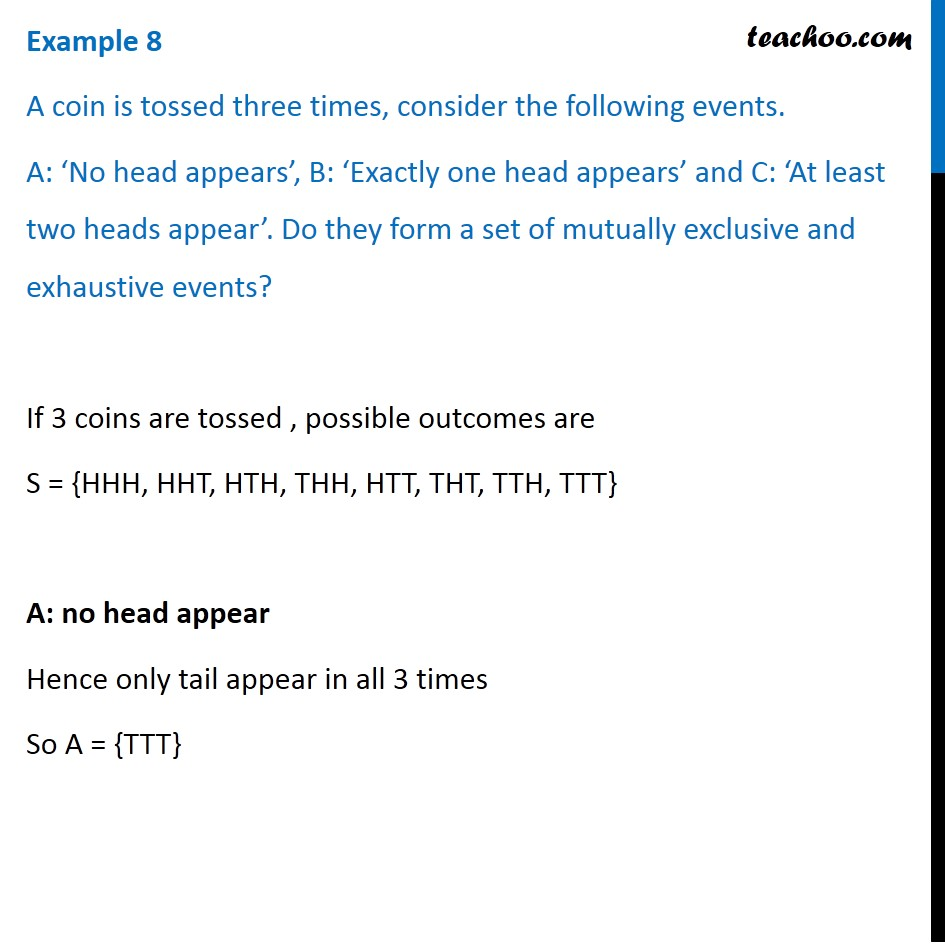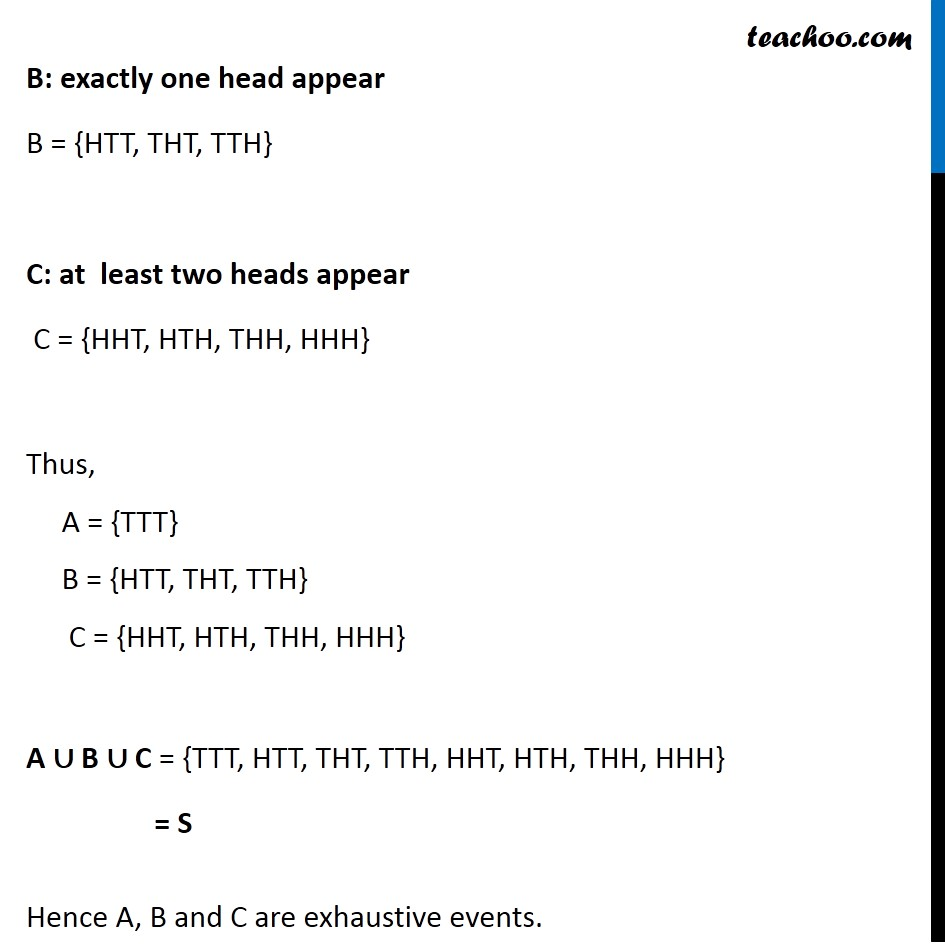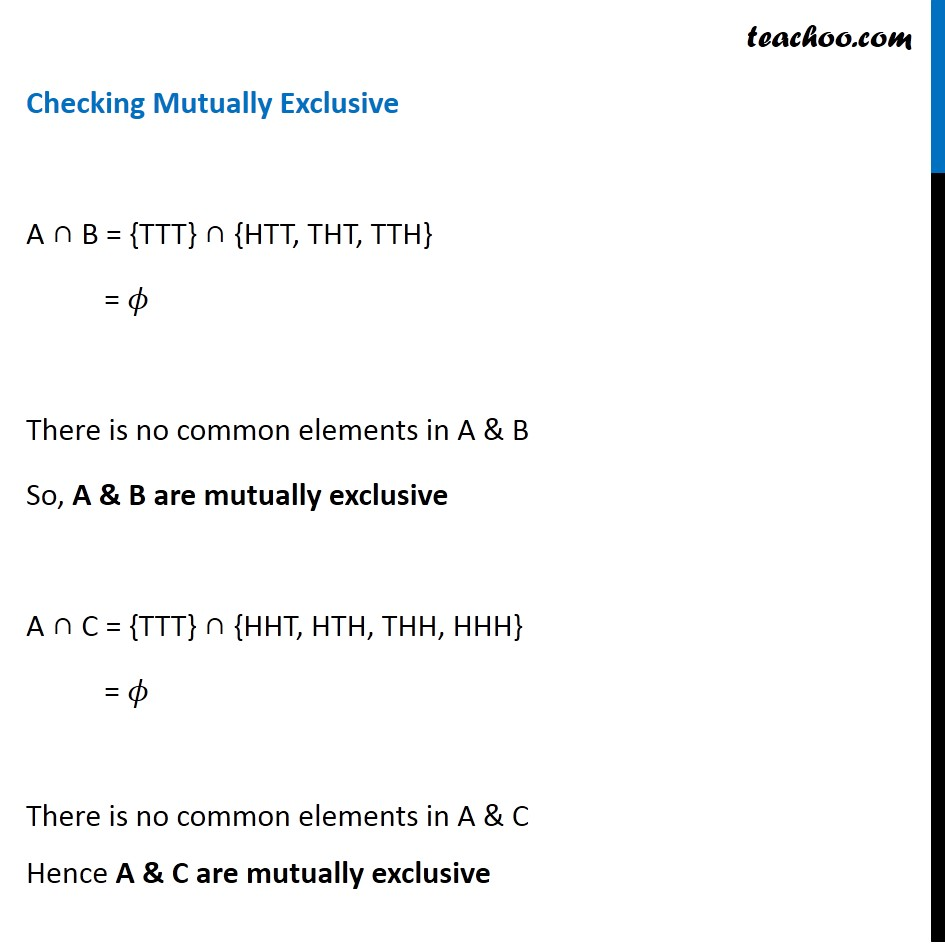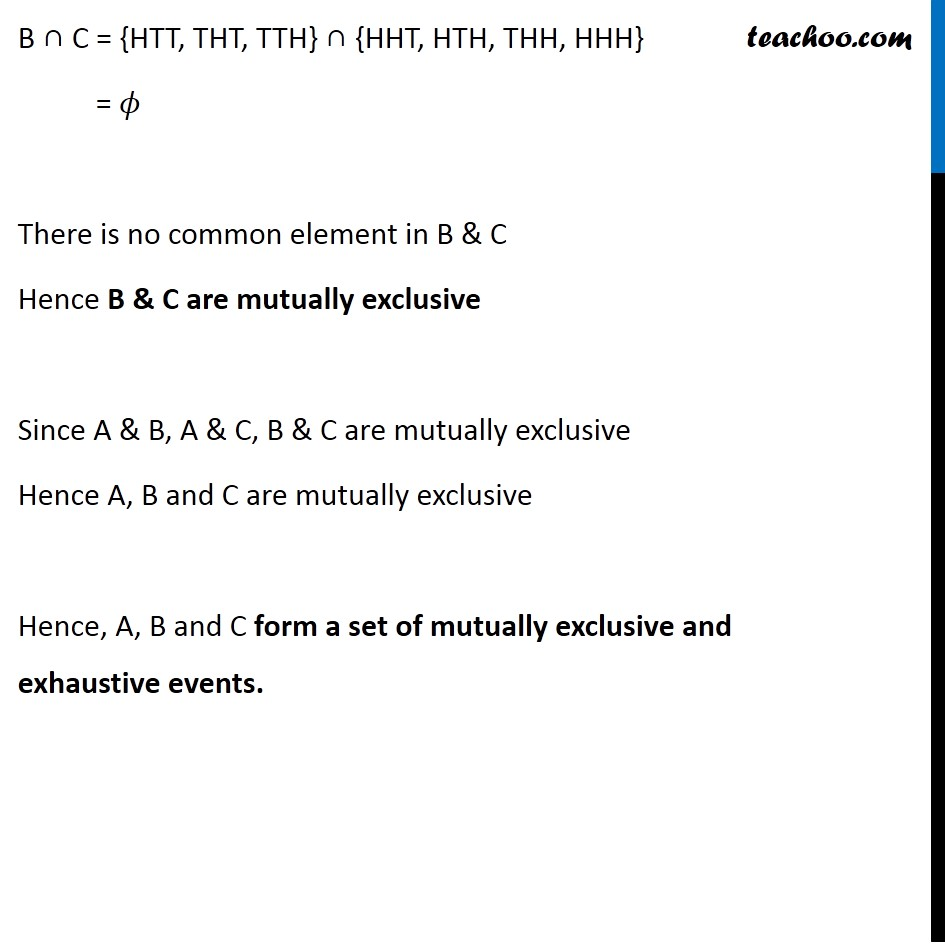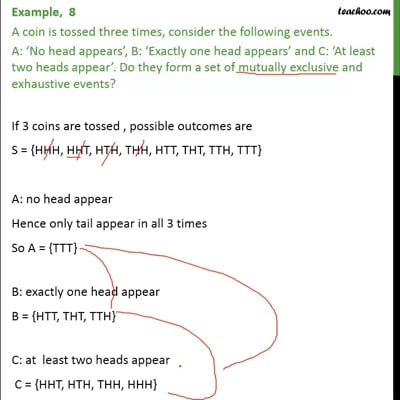This video is only available for Teachoo black users

Learn in your speed, with individual attention - Teachoo Maths 1-on-1 Class

### Transcript

Example 8 A coin is tossed three times, consider the following events. A: ‘No head appears’, B: ‘Exactly one head appears’ and C: ‘At least two heads appear’. Do they form a set of mutually exclusive and exhaustive events? If 3 coins are tossed , possible outcomes are S = {HHH, HHT, HTH, THH, HTT, THT, TTH, TTT} A: no head appear Hence only tail appear in all 3 times So A = {TTT} B: exactly one head appear B = {HTT, THT, TTH} C: at least two heads appear C = {HHT, HTH, THH, HHH} Thus, A = {TTT} B = {HTT, THT, TTH} C = {HHT, HTH, THH, HHH} A ∪ B ∪ C = {TTT, HTT, THT, TTH, HHT, HTH, THH, HHH} = S Hence A, B and C are exhaustive events. Checking Mutually Exclusive A ∩ B = {TTT} ∩ {HTT, THT, TTH} = 𝜙 There is no common elements in A & B So, A & B are mutually exclusive A ∩ C = {TTT} ∩ {HHT, HTH, THH, HHH} = 𝜙 There is no common elements in A & C Hence A & C are mutually exclusive B ∩ C = {HTT, THT, TTH} ∩ {HHT, HTH, THH, HHH} = 𝜙 There is no common element in B & C Hence B & C are mutually exclusive Since A & B, A & C, B & C are mutually exclusive Hence A, B and C are mutually exclusive Hence, A, B and C form a set of mutually exclusive and exhaustive events.